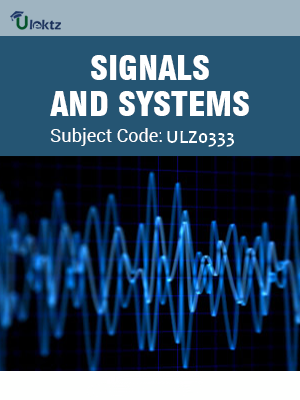•My WalletMy Order
•My Profile
•My Connections
•My Books
•My Videos
•My Tests
•My Calender
•My Messages
•My Shopping Cart
•My Orders
•Account Settings
•Help

# Book Details# Signals & Systems

 Course Code : ULZ0333 Author : uLektz University : General for All University Regulation : 2017 Categories : Electronics & Communication Format :ePUB3 (DRM Protected) Type : eBook

FREE

Description :Signals & Systems of ULZ0333 covers the latest syllabus prescribed by General for All University for regulation 2017. Author: uLektz, Published by uLektz Learning Solutions Private Limited.

Note : No printed book. Only ebook. Access eBook using uLektz apps for Android, iOS and Windows Desktop PC.

##### Topics
###### UNIT I SIGNALS AND SYSTEMS

1.1 Continuous-Time and Discrete-Time Signals,Transformations of the Independent Variable

1.2 Exponential and Sinusoidal Signals, the Unit Impulse and Unit Step Functions, Properties of impulse response representation

1.3 Continuous-Time and Discrete Time Systems, Basic System Properties, Linear Time-Invariant Systems, Discrete-Time LTI Systems, The Convolution Sum, Continuous-Time LTI Systems, The Convolution Integral

1.4 Properties of Linear Time-Invariant Systems,Causal LTI Systems Described by Differential and Difference Equations,Singularity Functions

###### UNIT II FOURIER SERIES REPRESENTATION OF PERIODIC SIGNALS

2.1 The Response of LTI Systems to Complex Exponentials

2.2 Fourier Series Representation of Continuous, Fourier series Analysis,Time Periodic Signals,Convergence of the Fourier Series, Properties of Continuous-Time Fourier Series

2.3 Fourier Series Representation of Discrete-Time Periodic Signals, Properties of Discrete-Time Fourier Series

2.4 Fourier Series and LTI Systems, Filtering - Examples of Continuous-Time Filters Described by Differential Equations

2.5 Examples of Discrete-Time Filters Described by Difference Equations

###### UNIT III THE CONTINUOUS-TIME AND DISCRETE-TIME FOURIER TRANSFORM

3.1 The Continuous-Time Fourier Transform: Representation of Aperiodic Signals, The Continuous- Time Fourier Transform,The Fourier Transform for Periodic Signals

3.2 Properties of the Continuous- Time Fourier Transform, The Convolution Property, Fourier Properties and Basic Fourier Transform Pairs, Systems characterized by Linear constant coefficient differential equations, The Discrete-Time Fourier Transform - Representation of Aperiodic Signals

3.3 The Discrete-Time Fourier Transform, The Convolution Property

3.4 Fourier Transform Properties and Basic Fourier Transform Pairs, Duality, Systems Characterized by Linear Constant-Coefficient Difference Equations

###### UNIT IV TIME & FREQUENCY CHARACTERIZATION OF SIGNALS AND SYSTEMS

4.1 The Magnitude-Phase Representation of the Fourier Transform, The Magnitude-Phase Representation of the Frequency Response of LTI Systems

4.2 Time-Domain Properties of Ideal Frequency-Selective Filters,Time- Domain and Frequency-Domain Aspects of Non-ideal Filters

4.3 First-Order and Second-Order Continuous-Time Systems, First-Order and Second-Order Discrete-Time Systems, Examples of Time and Frequency-Domain Analysis of Systems

4.4 Sampling: Representation of a Continuous-Time Signal by Its Samples, Sampling Theorem, Reconstruction of a Signal from Its Samples Using Interpolation

4.5 Effect of under sampling: Aliasing, Discrete-Time Processing of Continuous-Time Signals

###### UNIT V:Laplace and z-Transforms

5.1 The Laplace Transform, The Region of Convergence for Laplace Transforms, The Inverse Laplace Transform

5.2 Geometric Evaluation of the Fourier Transform from the Pole-Zero Plot, Properties of the Laplace Transform, Some Laplace Transform Pairs, Analysis and Characterization of LTI Systems Using the Laplace Transform, System Function Algebra and Block Diagram Representations

5.3 The Z-Transform, Region of Convergence for the z-Transform, The Inverse z-Transform, Geometric Evaluation of the Fourier Transform from the Pole-Zero Plot

5.4 Properties of the z-Transform, Some Common z-Transform Pairs,Analysis and Characterization of LTI Systems Using z-Transforms

5.5 System Function Algebra and Block Diagram Representations, Unilateral z-Transforms

### Related Books